# Boys and girls

There are eight boys and nine girls in the class. There were six children on the trip from this class. What is the probability that left

a) only boys
b) just two boys

Result

p1 =  0.226 %
p2 =  28.507 %

#### Solution:Leave us a comment of example and its solution (i.e. if it is still somewhat unclear...):

Showing 0 comments:Be the first to comment!#### To solve this example are needed these knowledge from mathematics:

Would you like to compute count of combinations? See also our permutations calculator. See also our variations calculator.

## Next similar examples:

1. ClassroomOf the 26 pupils in the classroom, 12 boys and 14 girls, four representatives are picked to the odds of being: a) all the girls b) three girls and one boy c) there will be at least two boys
2. Disco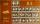On the disco goes 12 boys and 15 girls. In how many ways can we select four dancing couples?
3. DivisionDivision has 18 members: 10 girls and 6 boys, 2 leaders. How many different patrols can be created, if one patrol is 2 boys, 3 girls and 1 leader?
4. Hearts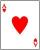5 cards are chosen from a standard deck of 52 playing cards (13 hearts) with replacement. What is the probability of choosing 5 hearts in a row?
5. TrinityHow many different triads can be selected from the group 43 students?
6. BallsFrom the urn in which are 7 white balls and 17 red, gradually drag 3-times without replacement. What is the probability that pulls balls are in order: red red red?
7. Theorem proveWe want to prove the sentense: If the natural number n is divisible by six, then n is divisible by three. From what assumption we started?
8. Permutations without repetitionFrom how many elements we can create 720 permutations without repetition?
9. Fish tankA fish tank at a pet store has 8 zebra fish. In how many different ways can George choose 2 zebra fish to buy?
10. Value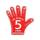Find the value of the expression: 6!·10^-3
11. CardsThe player gets 8 cards of 32. What is the probability that it gets a) all 4 aces b) at least 1 ace
12. One greenIn the container are 45 white and 15 balls. We randomly select 5 balls. What is the probability that it will be a maximum one green?
13. A pizzaA pizza place offers 14 different toppings. How many different three topping pizzas can you order?
14. Tokens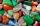In the non-transparent bags are red, white, yellow, blue tokens. We 3times pull one tokens and again returned it, write down all possibilities.
15. A bookA book contains 524 pages. If it is known that a person will select any one page between the pages numbered 125 and 384, find the probability of choosing the page numbered 252 or 253.
16. Calculation of CNCalculate: ?
17. The confectionery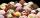The confectionery sold 5 kinds of ice cream. In how many ways can I buy 3 kinds if order of ice creams does not matter?